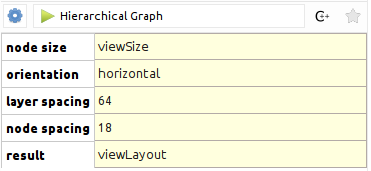# Applying an algorithm on a graph¶

In Tulip, algorithms are implemented through plugins (automatically loaded when importing the `tulip` module). There are two main type of algorithms:

• General: algorithms of this type can work on the input graph in a free manner and perform various tasks
(modifying the graph structure, creating graph properties, …).
• Property: algorithms of this type can only compute data to attach on the graph elements and fill an output
graph property (e.g. layout algorithm, metric algorithm, …).

In the remaining of this section, you will learn how to call each type of algorithm from Python but also how to transmit parameters to the algorithms.

## Calling a general algorithm on a graph¶

To call a general algorithm, you must use the `tlp.Graph.applyAlgorithm()` method. From the Tulip GUI, you can see all the general algorithms currently loaded by looking at the entries from the Algorithm -> General menu. Alternatively, from Python you can get a list of the general algorithms names by calling the `tlp.getAlgorithmPluginsList()` function.

As an example, we will call the “Equal value” algorithm from Python. This algorithm takes an input graph property and partitions the graph elements (nodes or edges) according to the values of the property. More precisely, all graph elements having the same property value are grouped in a class. Then for each class, a subgraph is created.

This algorithm takes 3 parameters as illustrated in the screenshot below:Below is a description of each parameter:

• Property: the input graph property of type `tlp.PropertyInterface` from which to compute the partition
• Type: a `tlp.StringCollection` parameter to choose the type of the elements to partition (two possible values : “nodes” or “edges”)
• Connected: a boolean parameter indicating whether the output subgraphs have to be connected

The sample code below illustrates how to call this algorithm from Python. We assume that a Tulip graph is bound to a “graph” variable and this graph contains a double property called “myMetric”:

```# get the input property from which to generate the partition
myMetric = graph.getDoubleProperty("myMetric")

# get a dictionary filled with default parameters for the algorithm
params = tlp.getDefaultPluginParameters("Equal Value", graph)

# set the input property
params["Property"] = myMetric

# if you want to partition edges instead of nodes
# params["Type"] = "edges"

# if you want connected subgraphs
# params["Connected"] = True

# now we call the algorithm
graph.applyAlgorithm("Equal Value", params)
```

## Calling a property algorithm on a graph¶

To call a property algorithm on a graph, you must use specific methods from the `tlp.Graph` class. There is one method per type of property to compute like for instance `tlp.Graph.applyBooleanAlgorithm()`, `tlp.Graph.applyLayoutAlgorithm()`, …

As an illustration, we will call the “Hierarchical Graph” layout algorithm, that can be used to draw a tree.

This algorithm takes 4 parameters as illustrated in the screenshot below:Below is a description of each parameter:

• node size: an input size property of type `tlp.SizeProperty` that contains nodes sizes
• orientation: a `tlp.StringCollection` parameter to choose the layout orientation (two possible values: “horizontal” or “vertical”)
• layer spacing: a float parameter to set up the minimum distance between two layers in the drawing
• node spacing: a float parameter to set up the minimum distance between two nodes in the same layer

The sample code below illustrates how to call this layout algorithm from Python. We assume that a Tulip graph is bound to a “graph” variable:

```# get a data set filled with default parameters for the algorithm
params = tlp.getDefaultPluginParameters("Hierarchical Graph", graph)

# set the orientation to vertical
params["orientation"] = "vertical"

# set custom spacing
params["layer spacing"] = 100.0
params["node spacing"] = 50.0

# get a reference to the default layout property
viewLayout = graph.getLayoutProperty("viewLayout")

# call the layout algorithm and store the result in viewLayout
graph.applyLayoutAlgorithm("Hierarchical Graph", viewLayout, params)
```